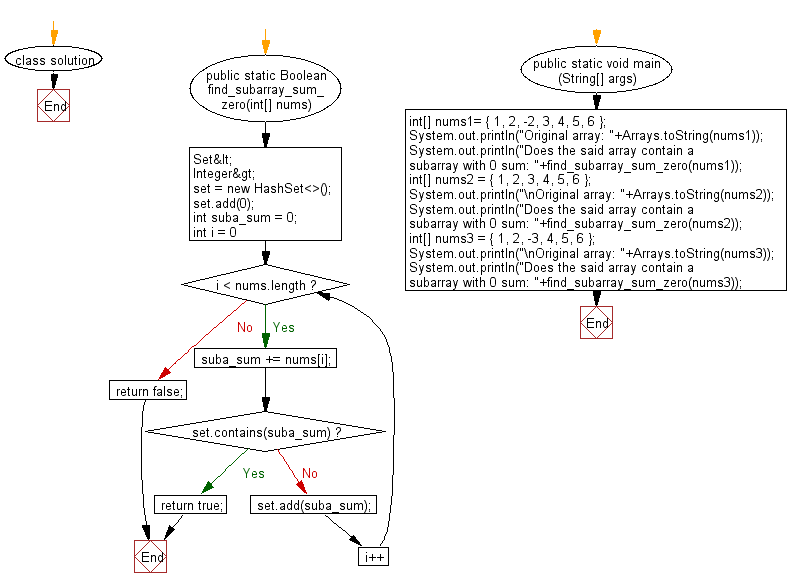﻿ Java: Check if a given array contains a subarray with 0 sum# Java Array Exercises: Check if a given array contains a subarray with 0 sum

## Java Array: Exercise-54 with Solution

Write a Java program to check if a given array contains a subarray with 0 sum.

Example:
Input :
nums1= { 1, 2, -2, 3, 4, 5, 6 }
nums2 = { 1, 2, 3, 4, 5, 6 }
nums3 = { 1, 2, -3, 4, 5, 6 }
Output:
Does the said array contain a subarray with 0 sum: true
Does the said array contain a subarray with 0 sum: false
Does the said array contain a subarray with 0 sum: true

Sample Solution:

Java Code:

``````import java.util.Set;
import java.util.HashSet;
import java.util.Arrays;

class solution
{
public static Boolean find_subarray_sum_zero(int[] nums)
{
Set<Integer> set = new HashSet<>();
int suba_sum = 0;
for (int i = 0; i < nums.length; i++)
{
suba_sum += nums[i];

if (set.contains(suba_sum)) {
return true;
}
}

return false;
}

public static void main (String[] args)
{
int[] nums1= { 1, 2, -2, 3, 4, 5, 6 };
System.out.println("Original array: "+Arrays.toString(nums1));
System.out.println("Does the said array contain a subarray with 0 sum: "+find_subarray_sum_zero(nums1));
int[] nums2 = { 1, 2, 3, 4, 5, 6 };
System.out.println("\nOriginal array: "+Arrays.toString(nums2));
System.out.println("Does the said array contain a subarray with 0 sum: "+find_subarray_sum_zero(nums2));
int[] nums3 = { 1, 2, -3, 4, 5, 6 };
System.out.println("\nOriginal array: "+Arrays.toString(nums3));
System.out.println("Does the said array contain a subarray with 0 sum: "+find_subarray_sum_zero(nums3));
}
}
```
```

Sample Output:

```
Original array: [1, 2, -2, 3, 4, 5, 6]
Does the said array contain a subarray with 0 sum: true

Original array: [1, 2, 3, 4, 5, 6]
Does the said array contain a subarray with 0 sum: false

Original array: [1, 2, -3, 4, 5, 6]
Does the said array contain a subarray with 0 sum: true
```

Flowchart:Java Code Editor:

Improve this sample solution and post your code through Disqus

What is the difficulty level of this exercise?

Test your Programming skills with w3resource's quiz.

﻿

## Java: Tips of the Day

getEnumMap

Converts to enum to Map where key is the name and value is Enum itself.

```public static <E extends Enum<E>> Map<String, E> getEnumMap(final Class<E> enumClass) {
return Arrays.stream(enumClass.getEnumConstants())
.collect(Collectors.toMap(Enum::name, Function.identity()));
}
```

Ref: https://bit.ly/3xXcFZt